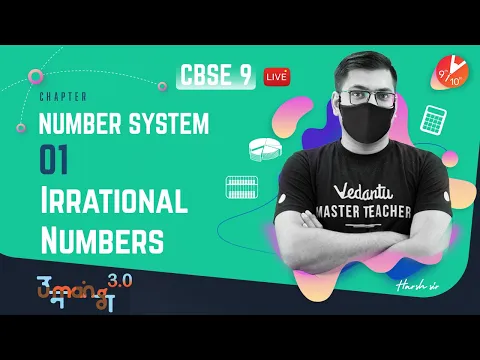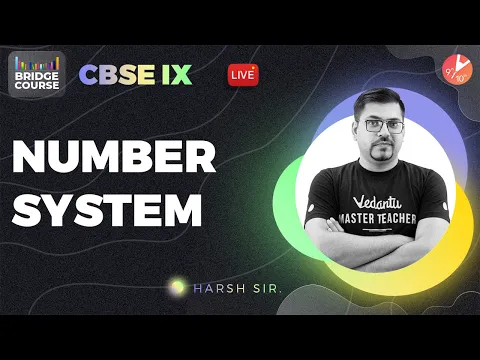Courses
Courses for Kids
Free study material
Free LIVE classes
More

# NCERT Books for Class 9 Maths Chapter 1LIVE
Join Vedantu’s FREE Mastercalss

## NCERT Books for Class 9 Maths - Number Systems - Free PDF Do.wnload

Free NCERT Books download for Class 9 Maths Chapter 1 - Number Systems on Vedantu. Students can also download the NCERT Book Solutions in PDF for Class 9 Maths. Register for Maths tuition to clear your doubts and score more in your exams. Subjects like Science, Maths, English will become easy to study if you have access to NCERT Solution Class 9 Science, Maths solutions, and solutions of other subjects that are available on Vedantu.

Last updated date: 27th Sep 2023
Total views: 601.8k
Views today: 17.01k
Watch videos on
NCERT Books for Class 9 Maths Chapter 1Number System in One Shot | CBSE Class 9 Maths Chapter 1 | CBSE lX - One Shot | Vedantu 9 and 10
Vedantu 9&10
SubscribeShare
11.5K likes
256.2K Views
2 years ago
Play QuizNumber System L-1 | Irrational Numbers | CBSE Class 9 Maths Chapter 1 | Umang 2021 | Vedantu 9 & 10
Vedantu 9&10
9K likes
186.5K Views
2 years agoNumber System | CBSE Class 9 Maths Chapter 1 - Bridge Course 🎯 | Harsh Sir | Vedantu 9 and 10
Vedantu 9&10
7.2K likes
143.8K Views
2 years ago

## How to download Class 9 Maths Chapter 1 NCERT Book for CBSE?

‘Number systems’ is the first chapter in the NCERT Maths textbooks for Class 9 students. In this chapter, students learn many concepts that are very important and build a foundation for the upcoming chapters. It begins with an introduction of the number line as well the different kinds of numbers on it such as natural numbers, whole numbers, integers and rational numbers. Students will get to learn how these numbers are denoted.  It is followed up by two other important topics on irrational numbers and real numbers.

A number is said to be irrational if it cannot be expressed in a ratio (p/q) where p and q are integers and q is not equal to 0. Further, students will learn about decimal expansions of real numbers and check whether it helps while differentiating between rational and irrational numbers; how to represent real numbers on the number line. They also learn about performing operations like addition, subtraction, multiplication, and division on irrational numbers. In the final section, students will learn about the laws of exponents for real numbers.

### Sections of Chapter 1- Number Systems

1.1 Introduction

1.2 Irrational Numbers

1.3 Real Numbers and their Decimal Expansions

1.4 Representing Real Numbers on the Number Line.

1.5 Operations on Real Numbers

1.6 Laws of Exponents for Real Numbers

1.7 Summary

### List of Exercises in Chapter 1- Number Systems

Exercise 1.1 - 4 questions

Exercise 1.2- 4 questions

Exercise 1.3- 9 questions

Exercise 1.4- 2 questions

Exercise 1.5- 5 questions

Exercise 1.6- 3 questions

### Advantages of referring to NCERT book for Class 9 Maths

The National Council of Educational Research and Training (NCERT), an autonomous organization, is known for designing textbooks for the primary as well as secondary levels. Let’s look at some of the features which distinguish NCERT textbooks from other books.

• The NCERT textbooks have numerous illustrations that make the textbook very interesting to study.

• After every concept is introduced, there are solved examples that solidify the concept in a student’s mind and add to their understanding.

• NCERT textbooks give students loads of practice as there is an exercise with different kinds of questions for every section in the chapter.

• These books are designed by subject matter experts and carefully researched.

• These books are very easy to follow. Each concept is explained in detail and followed with solved examples and exercises.

• NCERT textbooks adhere to the CBSE syllabus and are prescribed by CBSE themselves.

• These books are more than enough for preparing for the class 9 exam and are also used to prepare for competitive exams like JEE and NEET.

• NCERT Maths textbooks have a chapter summary in the end that is very helpful during revision.

## FAQs on NCERT Books for Class 9 Maths Chapter 1

1. Where can I find useful study resources for class 9 Maths?

You can find everything you need on the Vedantu app or website. These resources are created by professionals in the field and the content is accurate and reliable. Students can find revision notes, important questions, question papers, and a whole lot more! These study materials are available for free. All students have to do is sign up on the website and then they will be able to download content in pdf format. You can take advantage of these free resources to help you ace your exams.

2. Why is studying from the NCERT book essential for Class 9?

NCERT textbooks are prescribed by the CBSE board. These books follow the latest CBSE syllabus. Hence, these books are sufficient while preparing for Class 9 exams. It explains concepts in a clear and detailed manner. These textbooks are the best when it comes to strengthening one's fundamentals. It has numerous solved examples and exercises that add to a students’ learning. Hence, students are advised to study the NCERT book thoroughly.

3. What are the topics covered in chapter 1 of the Class 9 Maths NCERT textbook?

Chapter 1- Number systems begins with an introduction of number systems. There are different kinds of numbers on the number line such as natural numbers, whole numbers, integers, rational and irrational numbers. Students will further learn about real numbers and their decimal expansions. You will learn how to represent real numbers on the number line. You will study how to perform addition, subtraction, division, and multiplication on real numbers.  The last section deals with the laws of exponents for real numbers.

4. Is it necessary to solve all the chapter exercises?

It is always advisable to solve all the chapter exercises as these help you to practise your concepts. It requires hard work and practice. The NCERT textbook has examples and exercises after every section. After going through each section, go through the solved examples and understand them completely. Then move on to the exercises and try to solve them. There are chances of the same questions coming in the examination so students are advised to practice the sums in the exercises well.

5. How to score well in Class 9 Maths?

Practising maths is the only way to score well in class 9 Maths. Solve all the chapter exercises. This will improve your problem-solving ability and improve your speed and efficiency. You can make notes of important formulas, definitions, and equations in a notebook and revise them regularly. Solve sample papers and question papers of previous years within a time limit. This will familiarize you with the paper pattern, question type as well as improve your time management skills. Revise regularly so that you’re able to retain all you’ve studied for a longer period.